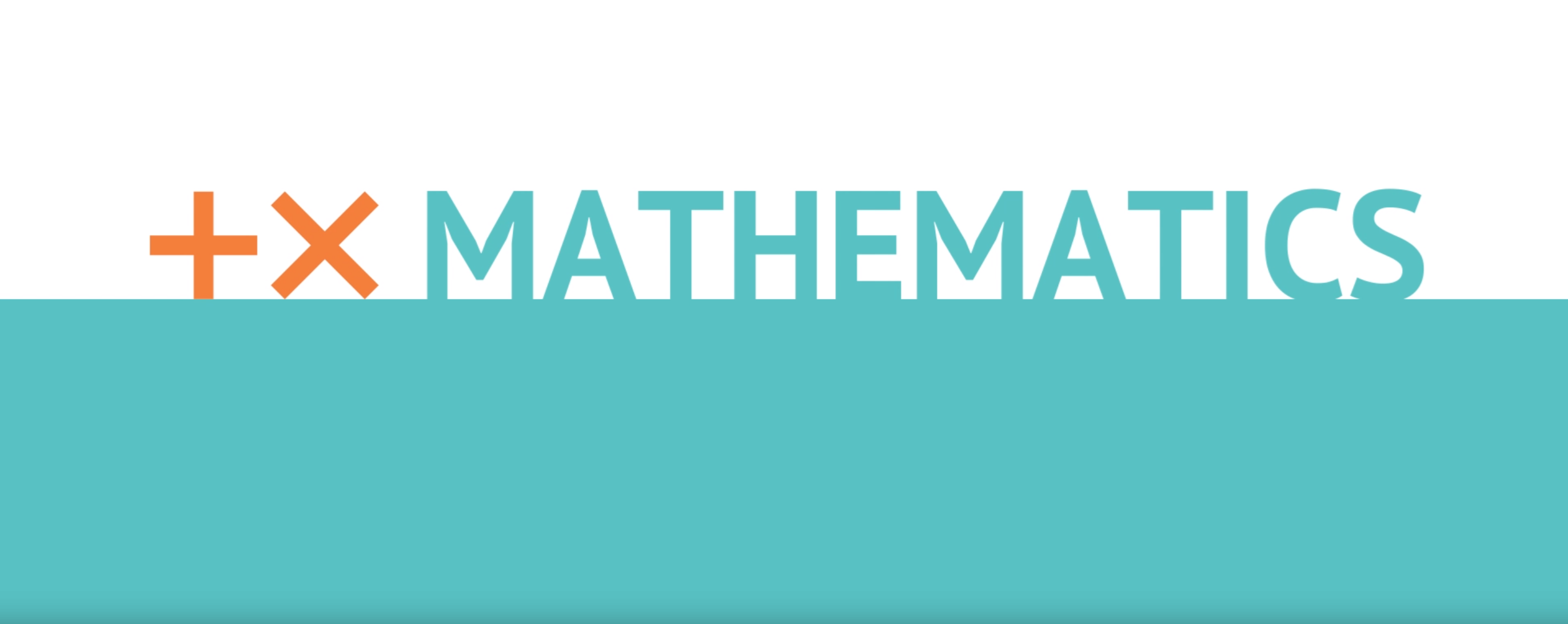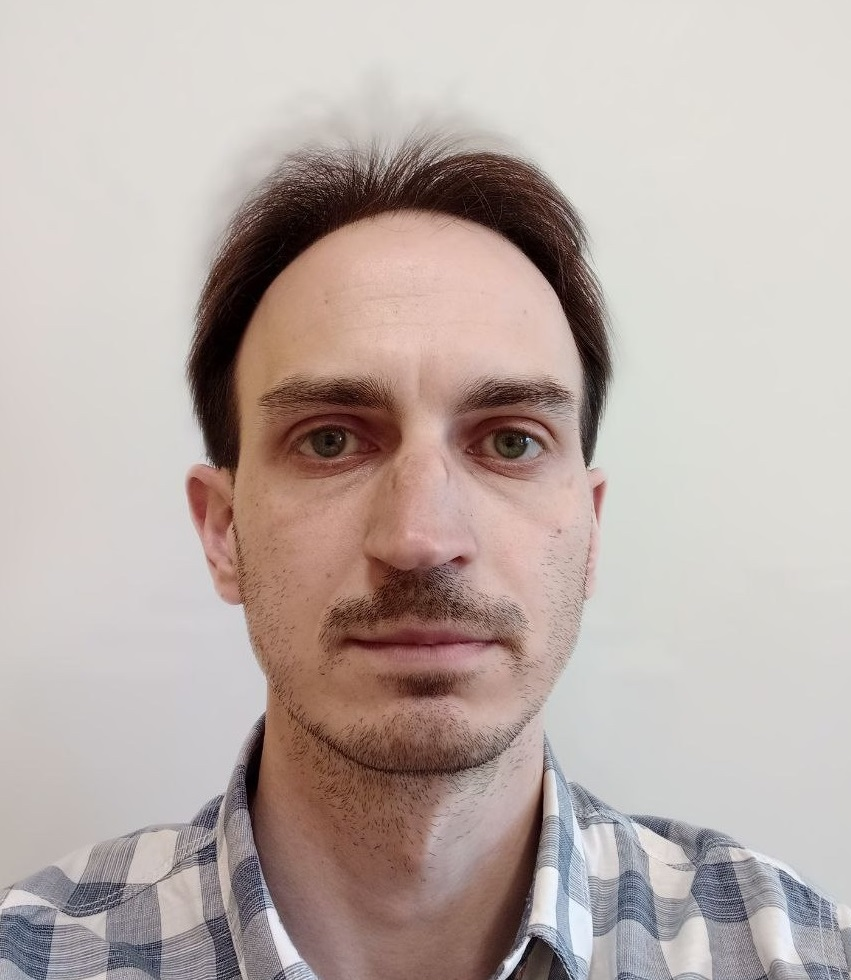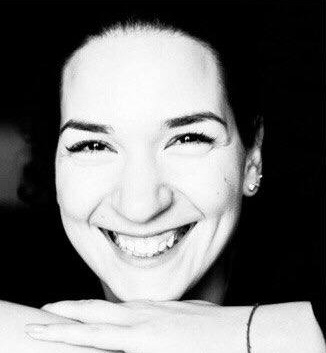# Mathematics

St. Petersburg Electrotechnical University 'LETI'
Enrollment in this course is by invitation onlyThe course is dedicated to studying the main theoretical concepts of algebra and their application to solving various types of tasks that occur in different fields of mathematics. The theoretical part of the course includes basic concepts of algebra, including rational and irrational polynomials, modulus, powers and logarithms, trigonometry, plane and analytic geometry. The practical part of the course includes examples of tasks corresponding to these themes, as well as the methods and techniques used to solve them. This knowledge and skills allow students to start successfully the university course in calculus.

The online course is developed for use in distance and blended learning.

## Objectives

• The study of basic concepts of algebra, including rational and irrational polynomials, modulus, powers and logarithms, trigonometric functions, plane and analytic geometry, progressions and vector operations.
• Formation of the ability to solve the most widespread types of problems related to these concepts.

## Common Course Outline

• Module 1. Algebraic equations, inequalities and systems
• Lesson 1.1 Basic concepts and definitions: the dictionary of algebra
• Lesson 1.2 Linear and quadratic equations
• Lesson 1.3 Systems of equations
• Lesson 1.4 Inequalities and systems of inequalitiess
• Module 2. Modulus (absolute value)
• Lesson 2.1 Definition of modulus
• Lesson 2.2 Equations including absolute values, and the method of intervals
• Module 3. Irrational equations and inequalities
• Lesson 3.1 Basic concepts: irrational numbers and functions. Radicals
• Lesson 3.2 Irrational equations. Extraneous roots
• Lesson 3.3 Irrational inequalities
• Module 4. Powers and logarithms
• Lesson 4.1 Concepts and basic properties of power and exponential function
• Lesson 4.2 Logarithms: concepts and basic properties
• Lesson 4.3 Exponential and logarithmic equations
• Module 5. Trigonometry
• Lesson 5.1 Main concepts: the trigonometric circle, measurement of angles, trigonometric functions
• Lesson 5.2 Basic trigonometric formulae
• Lesson 5.3 Trigonometric equations and inequalities
• Module 6. Arithmetic and geometric progressions
• Lesson 6.1 Definition and basic properties of progressions
• Lesson 6.2 Basic formulae and tasks concerning progressions
• Module 7. Plane geometry
• Lesson 7.1 Basic concepts: point and line, line segment, circle
• Lesson 7.2 Triangles: definition, special cases, properties and formulae
• Lesson 7.3 Quadrilaterals: definition, special cases, properties and formulae
• Module 8. Analytic geometry
• Lesson 8.1 Basic concepts: designation of points, lines and circles. Analytic representation of lines and circles
• Lesson 8.2 Conditions for parallel and intersecting lines. Examples of tasks in analytic geometry
• Module 9. Vector algebra
• Lesson 9.1 Basic concepts and definitions
• Lesson 9.2 Vector operations
• Module 10. Tasks containing parameters
• Lesson 10.1 The concept of parameter, forming a family of equations (inequalities)

For the attestation, the student should:

• pass entry quiz (10% of the final grade);
• pass control tests for the course modules (50% of the final grade);
• pass examination testing (30% of the final mark).

## Rating system

The results of tests and completed assignments are evaluated according to the rating system, the total amount of accrued interest for all types of activities is converted into an assessment on a four-point scale:

• «excellent» – at least 90% of successfully performed;
• «good» – at least 70%, less than 90%;
• «satisfactory» – at least 60%, less than 70%;
• «unsatisfactory» – less than 60%.

## Entry requirements and target audience

The course is designed for school students of pre-graduate years and bachelors of the 1st year of study.

If you pass an introduction test for this course you will be accepted on a course.

## Technical requirements

Review the technical requirements to access the course and complete it successfully.

## Course authors### Goryainov Victor Sergeevich

PhD, Assoсiate professor of Photonics Department

St.-Petersburg Electrotechnical University «LETI»### Zhikorentseva Polina Aleksandrovna

Assistant professor of Humanities and Bioethics Department

St.-Petersburg Pediatric Medical University
1. Course Number

Math101
2. Classes Start

3. Classes End

4. Estimated Effort

4 hour per week
5. Number of Weeks

16